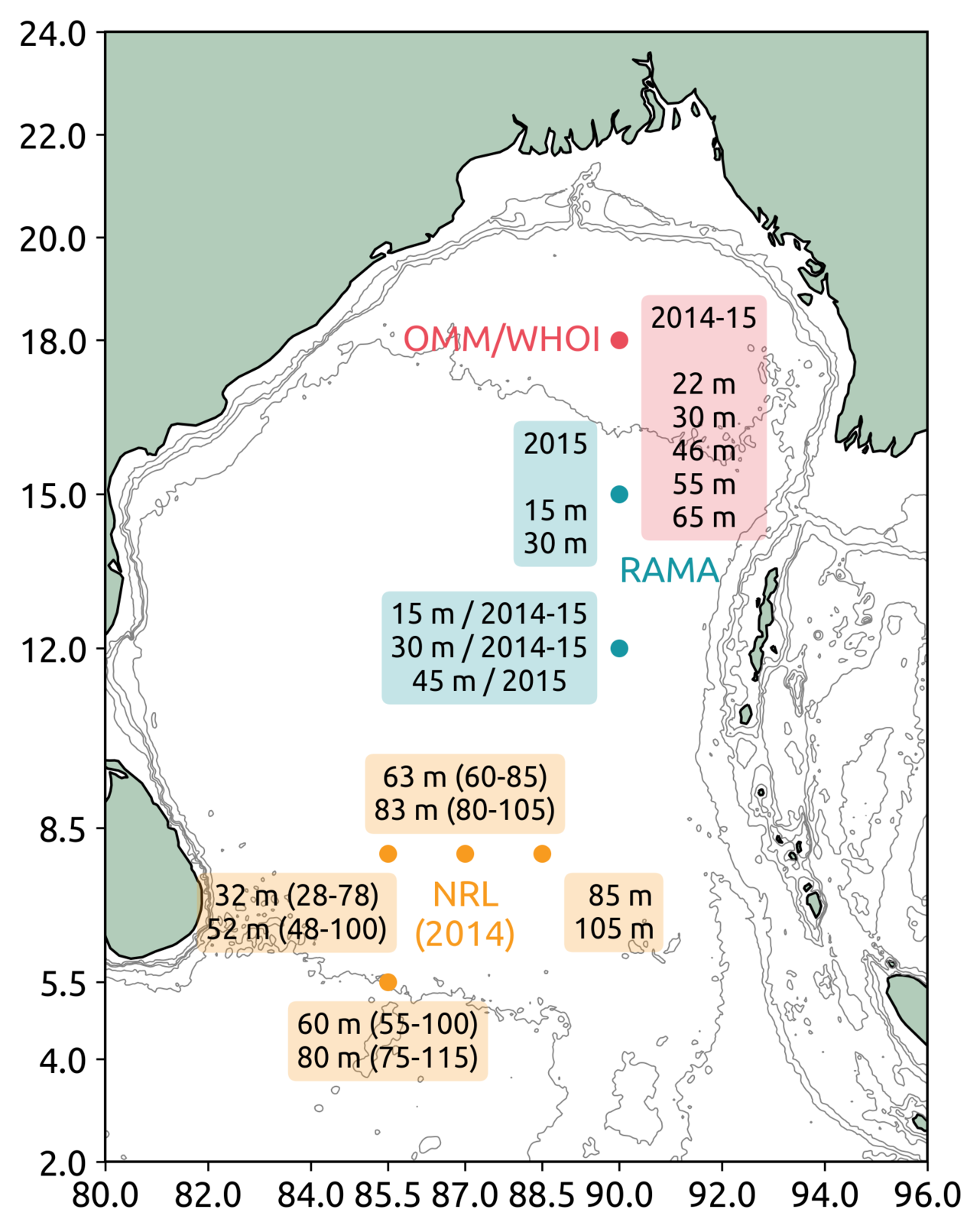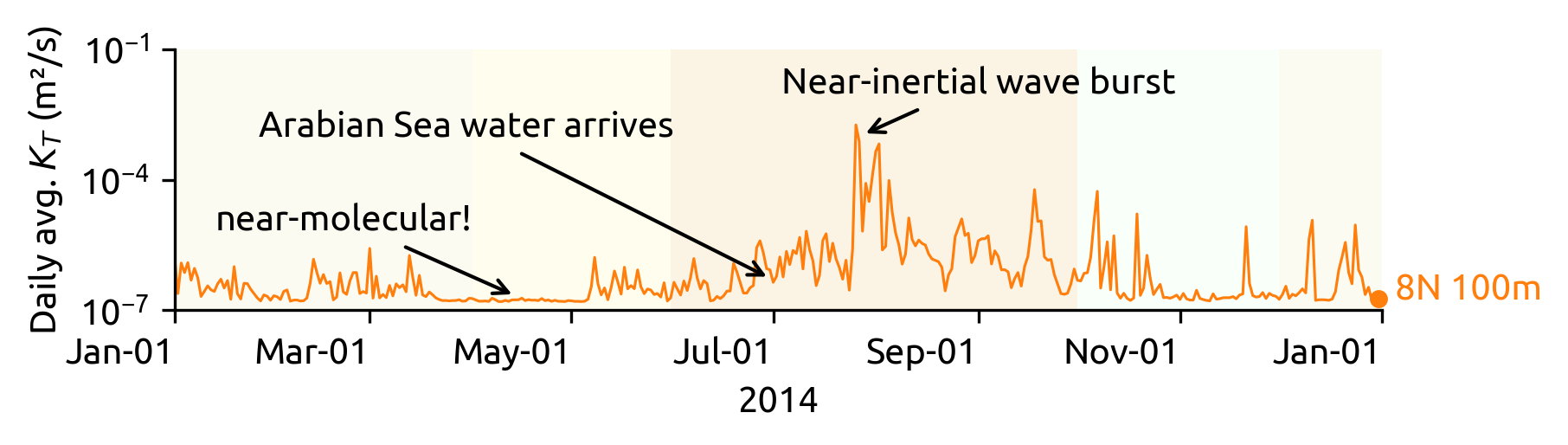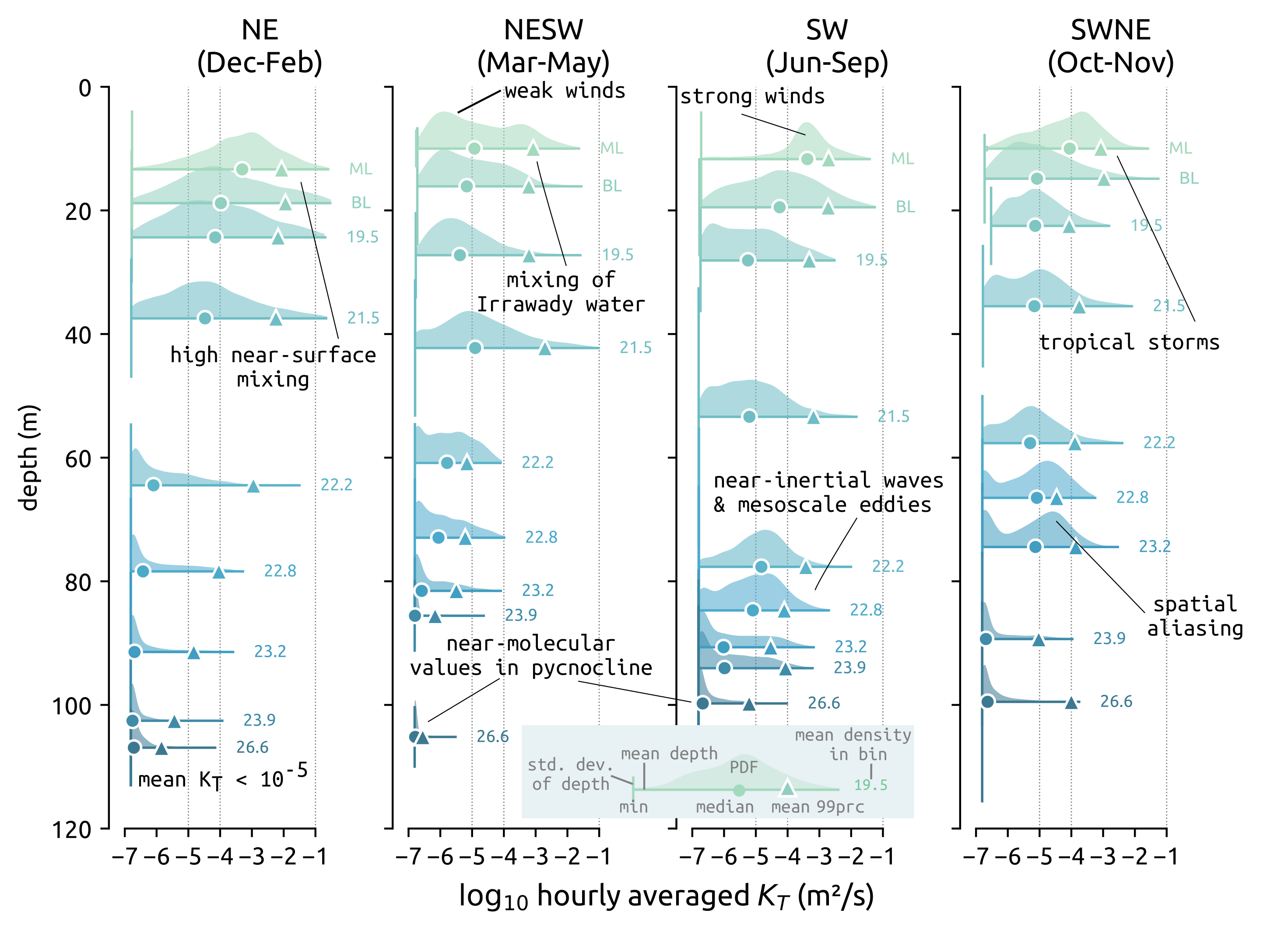← Back | Deepak Cherian | 18 Feb 2018

# Subsurface turbulent mixing in the Bay of Bengal

## MeasurementsFigure 1: Left: Locations and depths of χpods deployed in the Bay during 2014-15. The NRL moorings suffered some blowdown (depth ranges are indicated in parenthesis). Right: A χpod

For now I am ignoring the data at 18N. The map below consists of data from RAMA moorings at 12N, 15N and the NRL EBoB array.

## Specific examples

The most surprising signal is the near-molecular values observed in the thermocline (≤ 100m) at many locations in the SW Bay (off Sri Lanka).Figure 1: Time series of $$K_T$$ from NRL5 (88.5E, 8N) at approx. 100m. Note the near-molecular values in daily averaged $$K_T$$ during Apr-May.

It appears that strain values are a lot smaller than expected from the Garrett-Munk spectrum.

## $$K_T$$ maps

### Method

To combine data at different depths and locations throughout the Bay:

1. Label each $$K_T$$ estimate by whether it was made in the mixed layer ML or barrier layer BL, otherwise label it with the density of water parcel. In addition, label $$K_T$$ estimate with the depth at which that estimate was made.
2. Bin all observations in density space.
1. For each density bin, estimate a “mean depth” and standard deviation of observation depths. In addition, make a kernel density estimate which is one way to estimate a PDF.
3. Make a vertical profile of the PDFs:
1. The baseline of each PDF is located at the “mean depth”.
2. The extent of the baseline ranges from the minimum value observed to the 99th percentile.
3. The vertical bar at the left edge of the baseline spans one standard deviation above and below the “mean depth”. This gives us an impression of how much depth space is occupied by that isopycnal bin.
4. Means and medians are represented by triangles and circles respectively.

### Caveats

Binning in isopycnal space results in spatial aliasing — there is no guarantee that all moorings in an isopycnal bin witness the same mesoscale/internal-wave climate. One example is the double peaked distributions in the last column of the figure below. There are 3 moorings represented in these distributions: one mooring at (85.5E, 5N) consistently shows higher mixing than the other two — (87E, 8N) and (88.5E, 8N).

### $$K_T$$ summaryFigure 3: Seasonal variation of the vertical profile of $$K_T$$ distributions in the Bay. ML: mixed layer; BL: barrier layer. The numbers (e.g. 19.5) represent the mean density in that density bin. In this case 19.6 → 1019.6 kg/m³. Each column is a season; Dec-Feb means Dec 01-Feb 28.